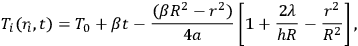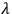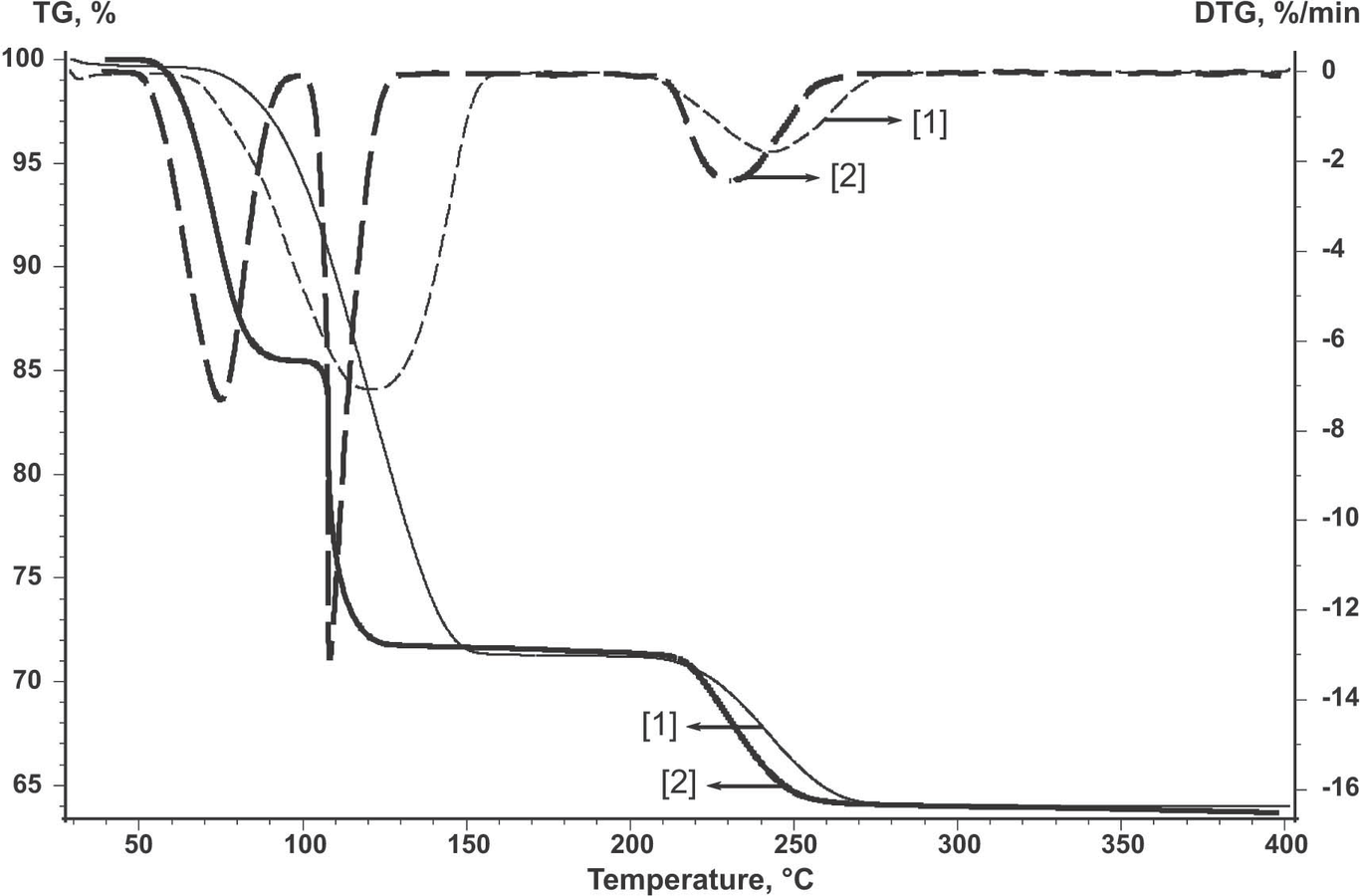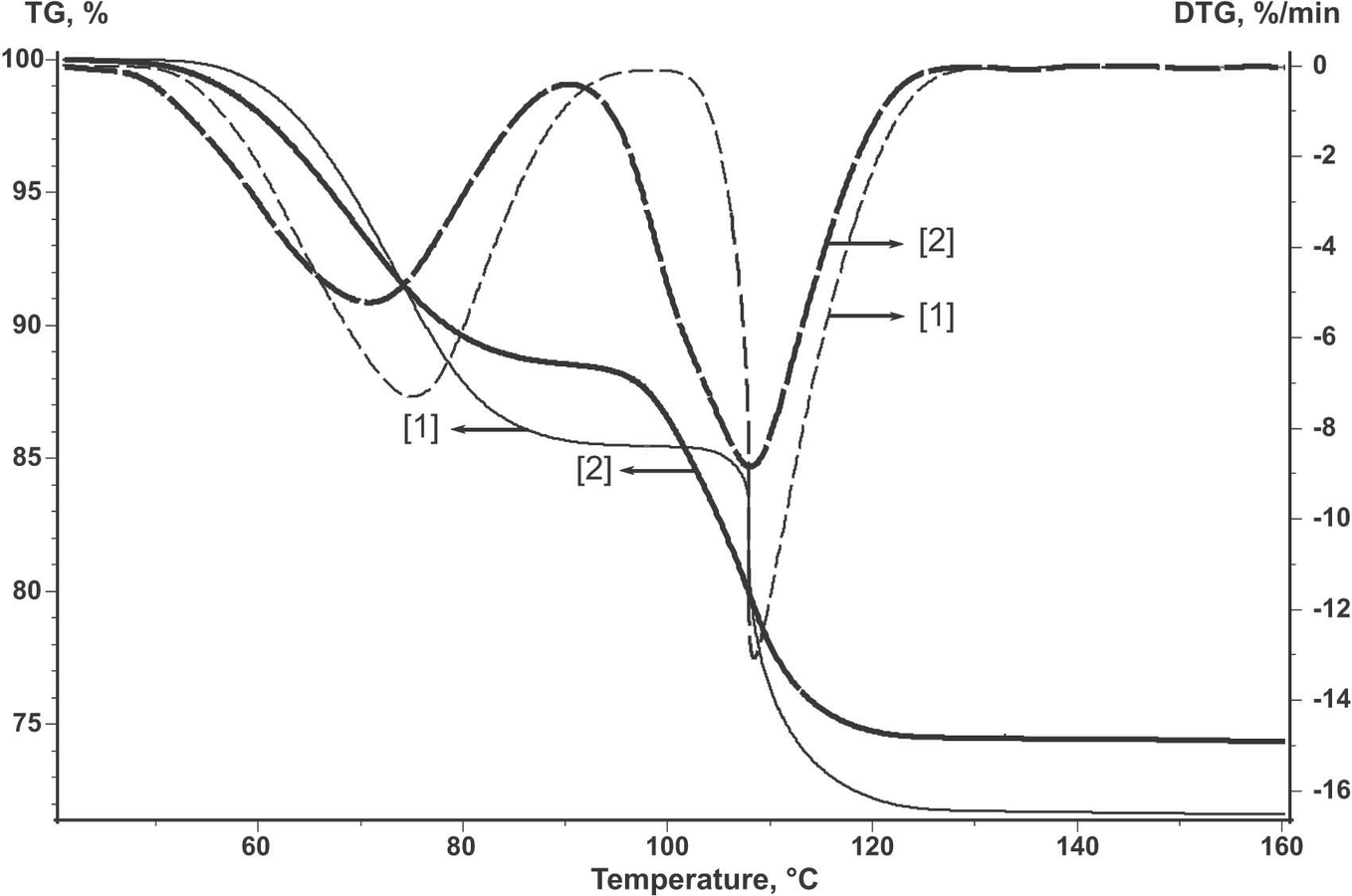# 2 Kinetic Experiment and Separation Methods

#### DOI

10.34663/9783945561171-04

#### Citation

Arhangel‘skii, Igor V., Dunaev, Alexander V., Makarenko, Irina V., Tikhonov, Nikolay A. and Tarasov, Andrey V. (2013). Kinetic Experiment and Separation Methods. In: Non-Isothermal Kinetic Methods: Workbook and Laboratory Manual. Berlin: Max-Planck-Gesellschaft zur Förderung der Wissenschaften.

## 2.1 Heat Transfer Conditions

As is known, the thermoanalytical experiment is carried out under variable temperature conditions, most frequently at a constant heating rate. Thereby, a so-called quasi-stationary temperature gradient appears in the bottom-heated sample. The temperature at every point of a thermally inert cylindrical sample with radius R and height H≤4R is described by the following equation:2.1

where Ti(ri,t) is the temperature at the ith point of the sample, T0 is the starting temperature of the experiment, β is the temperature change rate dT/dt = constant, t is time, R is the radius of the cylindrical sample, ri is the radius vector of a point of the sample, a is the thermal diffusivity,is the thermal conductivity, and h is the heat emission coefficient in the sample–holder system. Equation 2.1, which is an analytical representation of the solution to the heat transfer equation under certain assumptions, shows that a so-called quasi-stationary temperature regime is established in a sample, corresponding to a parabolic temperature field in the sample–holder system identical at any moment in time before the onset of thermal processes. Hence, the “conversion field” has the same shape, that is, each point of the sample is in its own state differerent from a neighboring one. Thus, different processes can occur at different points of the sample. In a chemical reaction accompanied by heat release or absorption (exo- and endothermic reactions), the temperature field can change significantly and temperature gradients can be as large as several tens of kelvins. To avoid this, conditions should be created under which the temperature gradients in the reacting system would not exceed the quasi-stationary gradient within the error of determination. This requirement is fulfilled under heat dilution conditions when the temperature field and heat exchange conditions are dictated by the thermophysical properties of the sample holder. This occurs in studying small amounts of a substance when the sample holder is made up of a metal with high heat conductance and its weight significantly exceeds the weight of the sample. Under these conditions, a so-called degenerate regime is realized, and heat exchange conditions have little effect on the kinetics of the process.

## 2.2 Mass Transfer Conditions

The mathematical description of mass transfer events accompanying heterogeneous processes is beyond the scope of this section. Rather, the aim is to show, at the qualitative level, how they can be experimentally affected. Let us consider the simplest heterogeneous process described by Equation 1.1.

In this process, several possible diffusion steps can be discerned. First, this is diffusion of gaseous products through the solid surface–environment interface. This mass transfer step can be controlled by purging the reaction volume with an inert gas. Figure 2.1 shows the results of studying the dehydration of CuSO4·5H2O. The curve reflecting a three-stage process was obtained under dynamic environment conditions. The air flow rate was 40 mL/min. The curve indicates the loss of five water molecules; the water is removed stepwise, two molecules at a time in 40–180°C region and the fifth molecule is released at 210–270°C.Fig. 2.1: Dehydration of CuSO4·5H2O in an open crucible in a dry air flow (curve 2) and in a static atmosphere (curve 1).

Fig. 2.1: Dehydration of CuSO4·5H2O in an open crucible in a dry air flow (curve 2) and in a static atmosphere (curve 1).

The TG curve of a two-stage process pertains to the process in a static atmosphere. Figure 2.1 demonstrates that the process in a static atmosphere has another mechanism as compared with the process in an open crucible in the air flow. It is believed that diffusion hindrances arising in a static atmosphere are responsible for a significant effect of the back reaction. Since the CuSO4·5H2O dehydration is a reversible process, its kinetics changes noticeably. If changing the flow rate does not change the process rate, this step of mass transfer has no effect on the overall rate.

Second, the mass transfer in the porous medium of the initial reagent is worthy of consideration. The simplest way to verify the significance of this step is to carry out a series of experiments with a sample of different thickness at the same external surface area. If the change in the layer height has no effect on the process rate, the diffusion in the porous reagent can be approximately considered to have little effect on the process as a whole.

The step of mass transfer in the layer of the solid reaction product is the hardest to identify. A possible way to reveal diffusion limitations at this step is to determine how the composition changes in different parts of the solid reagent at various depths. However, this procedure is rather laborious and necessitates the use of appropriate analytical methods and a special sample preparation. To determine the role of diffusion limitations in the product layer, it is common practice to compare the propagation kinetics of the interface measured at different conversions. If diffusion hindrances exist, the Arrhenius parameters decrease with an increase in the product layer thickness. If the temperature coefficient of the reaction rate E/R (in general case it is better to use E/R instead of E, because E is measured in J/mol while for thermoanalytical data of any material or blend using of “mol” unit is meaningless) remains constant at different conversions, it can be stated that this type of diffusion is not a rate-limiting stage.

Thus, diffusion hindrances can manifest themselves at different steps of the process under consideration and can depend on both the design of equipment and the nature of substances involved in the process.

A conclusion that can be drawn from the above is that to mitigate a noticeable effect of transfer processes of experimental results, small amounts of the initial reagent (a few milligrams) with minimal porosity or lower heating rates should be used. In addition, it is important that the sample is placed on a rather large surface and that purge gases at a rather high flow rate are used.

## 2.3 Nucleation

If our experiment is carried out under conditions such that transfer phenomena have no effect on the shape of thermoanalytical curves, the reaction can be thought of, to a first approximation, as a quasi-one-stage process representing the chemical transformations of reaction 1.1. However, the experimental results depend also on a change in the morphology of the initial reagent, i.e., on the formation of the reaction product, first of all, on the reagent surface. In this case, the conversion kinetics is dominated by the nucleation of the new phase and the subsequent growth of its nuclei. For heterogeneous processes, we are usually not aware of what atomic or molecular transformations lead to the nucleation of the product phase, so that the process is represented by a set of some formally geometric transformations. Non-isothermal kinetics is aimed at finding the forms of functions and their parameters describing these transformations.

Nucleation is related to the chemical stage of the process. However, because of the complexity and diversity of nucleation processes, we believe it is necessary to briefly dwell on this phenomenon, without going into theoretical descriptions of different steps of these processes. Consideration focuses on the manifestations of nucleation processes in the thermoanalytical experiment and on the proper design of the latter.

In the case of heterogeneous topochemical processes, the interface between the initial solid reagent and the solid reaction product is most frequently formed via nucleation processes. The reaction can simultaneously begin over the entire surface. In addition, nucleation can occur at separate sites of the surface, or by the branched chain mechanism, or another one. Possible mechanisms of these processes have been well documented (see, e.g., [1,4,5]). Here, we do not intend to go into details of all possible mechanisms; we will consider these phenomena in more detail when describing NETZSCH software.

For carrying out a kinetic experiment and obtaining reproducible results, it is necessary to standardize the surface of the initial reagent and create a definite amount of nuclei prior to the kinetic experiment. In the framework of thermoanalytical study, we can measure only the conversion (determination of the conversion or the overall reaction rate). Here, we do not consider the use of other physical methods for determining the number of nuclei on the reagent surface, for example, direct nucleus counting under the microscope. In thermal analysis, the most accessible and efficient method is natural nucleation under standard conditions. In this method, prior to the kinetic experiment, a noticeable amount of the initial reagent is heat treated up to a certain conversion. As a rule, the conversion amounts to several percent. The method is based on the fact that the last nucleation stages have little effect on the development of the reaction interface, since a large part of potential centers has already been activated. The sample thus standardized is used in all kinetic experiments, that is, at different heating rates.

Using the CuSO4·5H2O dehydration as an example, let us consider how the thermoanalytical curves change after natural nucleation under standard conditions as compared with the nonstandardized sample.

Figure 2.2 shows the TG and weight loss rate (DTG) curves for the initial untreated copper sulfate pentahydrate (curve 2) and for the sample subjected to natural nucleation under standard conditions (curve 1). To this end, the powder of the initial reagent was heated at T = 70 °C until 10% H2O was lost. As is seen, the shapes of the TG and DTG curves of the treated reagent differ from those of the initial reagent. Hence, the dehydration kinetics changes.Fig. 2.2: Dehydration of CuSO4·5H2O in an open crucible for the untreated sample (curve 2) and the standardized sample (curve 1).

Fig. 2.2: Dehydration of CuSO4·5H2O in an open crucible for the untreated sample (curve 2) and the standardized sample (curve 1).

Thus, using non-isothermal kinetics methods necessitates carrying out a special experiment involving a series of runs at various heating rates using the methods of separation of the rate-limiting stage, and small amounts of the solid reagent, purge gases, crucibles of appropriate size, and so forth.